# Difference between revisions of "0708-1300/Class notes for Thursday, January 17"

Announcements go here

Van-Kampen's Theorem

Let X be a point pointed topological space such that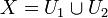$X = U_1\cup U_2$ where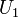$U_1$ and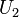$U_2$ are open and the base point b is in the (connected) intersection.

Then,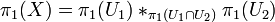$\pi_1(X) = \pi_1(U_1)*_{\pi_1(U_1\cap U_2)}\pi_1(U_2)$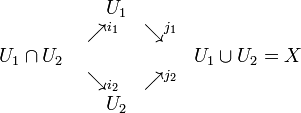$\begin{matrix} &\ \ \ \ U_1&&\\ &\nearrow^{i_1}&\searrow^{j_1}&\\ U_1\cap U_2&&&U_1\cup U_2 = X\\ &\searrow_{i_2}&\nearrow^{j_2}&\\ &\ \ \ \ U_2&&\\ \end{matrix}$

where all the i's and j's are inclusions.

Lets consider the image of this under the functor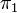$\pi_1$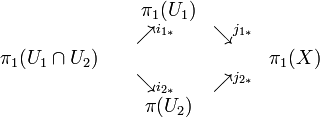$\begin{matrix} &\ \ \ \ \pi_1(U_1)&&\\ &\nearrow^{i_{1*}}&\searrow^{j_{1*}}&\\ \pi_1(U_1\cap U_2)&&& \pi_1(X)\\ &\searrow_{i_{2*}}&\nearrow^{j_{2*}}&\\ &\ \ \ \ \pi(U_2)&&\\ \end{matrix}$

Now consider the situation as groups: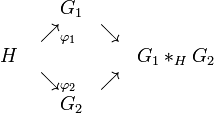$\begin{matrix} &\ \ \ \ G_1&&\\ &\nearrow_{\varphi_1}&\searrow&\\ H&&&G_1*_H G_2\\ &\searrow_{\varphi_2}&\nearrow&\\ &\ \ \ \ G_2&&\\ \end{matrix}$

Where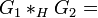$G_1 *_H G_2 =${ words with letters alternating between being in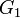$G_1$ and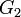$G_2$, ignoring e } / See Later

Considering just the set without the identification, we note this is a group with the operation being concatenation of words followed by reduction.

Ex: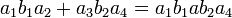$a_1b_1a_2 + a_3b_2a_4 = a_1b_1ab_2a_4$ where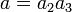$a = a_2a_3$

Claim:

This is really a group.

So far, we have only defined the "free group of$G_1$ and$G_2$". We now consider the identification (denoted above by 'See Later') which is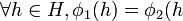$\forall h\in H, \phi_1(h) = \phi_2(h$)

With this identification we have properly defined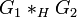$G_1 *_H G_2$

Note:$G_1 *_H G_2$ is equivalent to { words in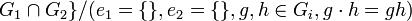$G_1\cap G_2\}/ (e_1 = \{\}, e_2 = \{\}, g,h\in G_i, g\cdot h = gh)$

Example 0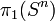$\pi_1(S^n)$ for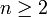$n\geq 2$

We can think of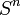$S^n$ as the union of two slightly overlapping open hemispheres which leaves the intersection as a band about the equator. As long as$n\geq 2$ this is connected (but fails for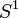$S^1$)

So,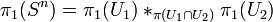$\pi_1(S^n) = \pi_1(U_1)*_{\pi(U_1\cap U_2)}\pi_1(U_2)$

But, since the hemispheres themselves are contractible,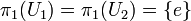$\pi_1(U_1) = \pi_1(U_2) = \{e\}$

Hence,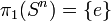$\pi_1(S^n) = \{e\}$

Example 1

Let us consider$\pi_1$ of a a figure eight. Let$U_1$ denote everything above a line slightly beneath the intersection and$U_2$ everything below a line slightly above the intersection point.

Now both$U_1$ and$U_2$ are homotopically equivalent to a loop and so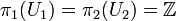$\pi_1(U_1) = \pi_2(U_2) = \mathbb{Z}$. We can think of these being the groups generated by a loop going around once, I.e., isomorphic to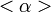$<\alpha>$ and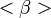$<\beta>$ respectively.

The intersection is an X, contractible to a point and so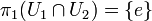$\pi_1(U_1\cap U_2) = \{e\}$

So$\pi_1$(figure 8)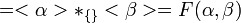$= <\alpha>*_{\{\}}<\beta> = F(\alpha,\beta)$ the free group generated by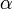$\alpha$ and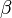$\beta$

This is non abelian

Example 2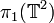$\pi_1(\mathbb{T}^2)$

We consider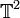$\mathbb{T}^2$ in the normal way as a square with the normal identifications on the sides. We then consider two concentric squares inside this and define$U_1$ as everything inside the larger square and$U_2$ as everything outside the smaller square.

Clearly$U_1$ is contractible, and hence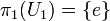$\pi_1(U_1) = \{e\}$

Now, the intersection of$U_1$ and$U_2$ is equivalent to an annulus and so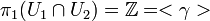$\pi_1(U_1\cap U_2) = \mathbb{Z} = <\gamma>$ where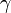$\gamma$ is just a loop in the annulus.

Now considering$U_2$, we note that each of the four outer corner points in the big square are identified, and when we identify edges we are left with something equivalent to a figure 8.

Hence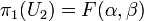$\pi_1(U_2) = F(\alpha, \beta)$ as in example 1

Hence,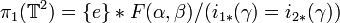$\pi_1(\mathbb{T}^2) = \{e\}*F(\alpha,\beta)/(i_{1*}(\gamma) = i_{2*}(\gamma))$

Now,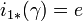$i_{1*}(\gamma) = e$

and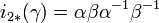$i_{2*}(\gamma) = \alpha\beta\alpha^{-1}\beta^{-1}$

I.e.,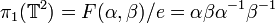$\pi_1(\mathbb{T}^2) = F(\alpha,\beta)/ e = \alpha\beta\alpha^{-1}\beta^{-1}$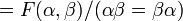$= F(\alpha,\beta)/(\alpha\beta = \beta\alpha)$

This is just the Free Abelian group on two symbols and,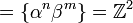$= \{\alpha^n\beta^m\} = \mathbb{Z}^2$

Hence,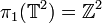$\pi_1(\mathbb{T}^2) = \mathbb{Z}^2$

Example 3

The two holed torus: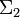$\Sigma_2$

Consider the schematic for this surface, consising of an octagon with edges labeled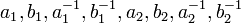$a_1,b_1,a_1^{-1},b_1^{-1},a_2,b_2,a_2^{-1},b_2^{-1}$

As in the previous example, consider two concentric circles inside the octagon. Let everything inside the larger circle be$U_1$ and everything outside the smaller circle be$U_2$.

Clearly$\pi_1(U_1) = \{e\}$ as before.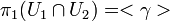$\pi_1(U_1\cap U_2) = <\gamma>$ as before.

Now,$U_2$ this times when doing the identifications looks like a clover (4 loops intersecting at one point)

Completely analogously to before, we see that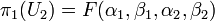$\pi_1(U_2) = F(\alpha_1, \beta_1, \alpha_2, \beta_2)$

Again,$i_{1*}(\gamma) = e$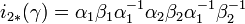$i_{2*}(\gamma) = \alpha_1\beta_1\alpha_1^{-1}\alpha_2\beta_2\alpha_1^{-1}\beta_2^{-1}$

Therefore,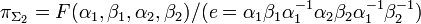$\pi_{\Sigma_2} = F(\alpha_1, \beta_1, \alpha_2, \beta_2)/(e =\alpha_1\beta_1\alpha_1^{-1}\alpha_2\beta_2\alpha_1^{-1}\beta_2^{-1})$

The abelianization of this group is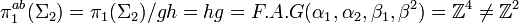$\pi_1^{ab}(\Sigma_2) = \pi_1(\Sigma_2)/ gh=hg = F.A.G (\alpha_1,\alpha_2,\beta_1,\beta^2) = \mathbb{Z}^4 \neq \mathbb{Z}^2$

In case someone might want diagrams for the examples above: